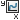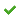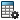Plotting the Kinetic Energy of a Part

You can plot the kinetic energy of a moving part.

1. From a Motion Analysis study, click Results and Plots(MotionManager toolbar).
2. In the PropertyManager, under Result, for Category, select Momentum/Energy/Power.
3. For Subcategory select from the following:
• Translational Kinetic Energy to calculate the kinetic energy due to the translational or linear motion of a part.
• Angular Kinetic Energy to calculate the kinetic energy due to the rotational motion of a part.
• Total Kinetic Energy to calculate the total kinetic energy due to the motion of a part.
4. Select the Plot Results options you require, and click.
The result appears in the MotionManager tree.
5. Click Calculate(MotionManager toolbar).